# Positive solutions of Sturm-Liouville boundary value problems for singular nonlinear second-order impulsive integro-differential equation in Banach spaces

## Abstract

In this work, we investigate the existence of positive solutions of Sturm-Liouville boundary value problems for singular nonlinear second-order impulsive integro differential equation in a real Banach space. Some new existence results of positive solutions are established by applying fixed-point index theory together with comparison theorem. Some discussions and an example are given to demonstrate the applications of our main results.

MSC:34B15, 34B25, 45J05.

## 1 Introduction

In this paper, we study the existence of positive solutions to second-order singular nonlinear impulsive integro-differential equation of the form:

${ y ′ ′ + h ( t ) f ( t , y ( t ) , y ′ ( t ) , ( T y ) ( t ) , ( S y ) ( t ) ) = 0 , ∀ t ∈ J , t ≠ t k , Δ y | t = t k = I k ( y ( t k ) ) , k = 1 , … , m , − Δ y ′ | t = t k = I ¯ k ( y ( t k ) , y ′ ( t k ) ) , k = 1 , … , m , α y ( 0 ) − β y ′ ( 0 ) = 0 , γ y ( 1 ) + δ y ′ ( 1 ) = 0 ,$
(1.1)

where $α,β,γ,δ≥0$, $ρ=βγ+αγ+αδ>0$, $I=[0,1]$, $J=(0,1)$, $0< t 1 < t 2 <⋯< t m <1$, $J ′ =J∖{ t 1 , t 2 ,…, t m }$, $J ¯ =[0,1]$, $J 0 =(0, t 1 ]$, $J k =( t k , t k + 1 ]$, $k=1,…,m−1$, $J m =( t m ,1]$, $f∈C[J×P×P×P×P,P]$, and P is a positive cone in E. θ is a zero element of E, $I k ∈C[P,P]$, $I ¯ k ∈C[P,P]$, and

$(Ty)(t)= ∫ 0 t K(t,s)y(s)ds,(Sy)(t)= ∫ 0 1 H(t,s)y(s)ds,$
(1.2)

in which $K∈C[D,J]$, $D={(t,s)∈J×J:t≥s}$, $H∈C[J×J,J]$, and $K 0 =max{K(t,s):(t,s)∈D}$, $H 0 =max{H(t,s):(t,s)∈D}$. $Δy | t = t k$ and $Δ y ′ | t = t k$ denote the jump of $y(t)$ and $y ′ (t)$ at $t= t k$, i.e.,

$Δy | t = t k =y ( t k + ) −y ( t k − ) ,Δ y ′ | t = t k = y ′ ( t k + ) − y ′ ( t k − ) ,$

where $y( t k + )$, $y ′ ( t k + )$ and $y( t k − )$, $y ′ ( t k − )$ represent the right-hand limit and left-hand limit of $y(t)$ and $y ′ (t)$ at $t= t k$, respectively. $h∈C(J, R + )$ and may be singular at $t=0$ and/or $t=1$.

Boundary value problems for impulsive differential equations arise from many nonlinear problems in sciences, such as physics, population dynamics, biotechnology, and economics etc. (see [1, 2, 414, 1618]). As it is well known that impulsive differential equations contain jumps and/or impulses which are main characteristic feature in computational biology. Over the past 15 years, a significant advance has been achieved in theory of impulsive differential equations. However, the corresponding theory of impulsive integro-differential equations in Banach spaces does not develop rapidly. Recently, Guo  established the existence of a solution, multiple solutions and extremal solutions for nonlinear impulsive integro-differential equations with nonsingular argument in Banach spaces. The main tools of Guo  are the Schauder fixed-point theorem, fixed-point index theory, upper and lower solutions together with the monotone iterative technique, respectively. The conditions of the Kuratowski measure of non-compactness in Guo  play an important role in the proof of the results. But all kinds of compactness type conditions is difficult to verify in abstract spaces. As a result, it is an interesting and important problem to remove or weak compactness type conditions.

Inspired and motivated greatly by the above works, the aim of the paper is to consider the existence of positive solutions for the boundary value problem (1.1) under simpler conditions. The main results of problem (1.1) are obtained by making use of fixed-point index theory and fixed-point theorem. More specifically, in the proof of these theorems, we construct a special cone for strict set contraction operator. Our main results in essence improve and generalize the corresponding results of Guo . Moreover, our method is different from those in Guo .

The rest of the paper is organized as follows: In Section 2, we present some known results and introduce conditions to be used in the next section. The main theorem formulated and proved in Section 3. Finally, in Section 4, some discussions and an example for singular nonlinear integro-differential equations are presented to demonstrate the application of the main results.

## 2 Preliminaries and lemmas

In this section, we shall state some necessary definitions and preliminaries results.

Definition 2.1 Let E be a real Banach space. A nonempty closed set $P⊂E$ is called a cone if it satisfies the following two conditions:

1. (1)

$x∈P$, $λ>0$ implies $λx∈P$;

2. (2)

$x∈P$, $−x∈P$ implies $x=0$.

A cone is said solid if it contains interior points, $P ∘ ≠θ$. A cone P is called to be generating if $E=P−P$, i.e., every element $y∈E$ can be represented in the form $y=x−z$, where $x,z∈P$. A cone P in E induces a partial ordering in E given by $u≤v$ if $v−u∈P$. If $u≤v$ and $u≠v$, we write $u; if cone P is solid and $v−u∈ P ∘$, we write $u≪v$.

Definition 2.2 A cone $P⊂E$ is said to be normal if there exists a positive constant N such that $∥x+y∥≥N$, $∀x,y∈P$, $∥x∥=1$, $∥y∥=1$.

Definition 2.3 Let E be a metric space and S be a bounded subset of E. The measure of non-compactness $ϒ(S)$ of S is defined by

Definition 2.4 An operator $B:D⟶E$ is said to be completely continuous if it is continuous and compact. B is called a k-set-contraction ($k≥0$) if it is continuous, bounded and $ϒ(B(S))≤kϒ(S)$ for any bounded set $S⊂D$, where $ϒ(S)$ denotes the measure of noncompactness of S.

A k-set-contraction is called a strict-set contraction if $k<1$. An operator B is said to be condensing if it is continuous, bounded, and $ϒ(B(S))<ϒ(S)$ for any bounded set $S⊂D$ with $ϒ(S)>0$.

Obviously, if B is a strict-set contraction, then B is a condensing mapping, and if operator B is completely continuous, then B is a strict-set contraction.

It is well known that $y∈ C 2 (0,1)∩C[0,1]$ is a solution of the problem (1.1) if and only if $x∈C[0,1]$ is a solution of the following nonlinear integral equation:

$y ( t ) = ∫ 0 1 G ( t , s ) h ( s ) f ( s , y ( s ) , y ′ ( s ) , ( T y ) ( s ) , ( S y ) ( s ) ) d s + ∑ 0 < t k < t [ I k ( y ( t k ) ) − ( t − t k ) I ¯ k ( y ( t k ) , y ′ ( t k ) ) ] + 1 ρ ( α t + β ) { ∑ k = 1 m [ γ ( 1 − t k ) I ¯ k ( y ( t k ) , y ′ ( t k ) ) + δ I ¯ k ( y ( t k ) , y ′ ( t k ) ) − γ I k ( y ( t k ) ) ] } ,$

where

$G(t,s)= 1 ρ { ( γ + δ − γ t ) ( β + α s ) , 0 ≤ s ≤ t ≤ 1 , ( β + α t ) ( γ + δ − γ s ) , 0 ≤ t ≤ s ≤ 1 ,$
(2.1)

where $ρ=γβ+αγ+αδ>0$. In what follows, we write $J 1 =[0, t 1 ]$, $J k =( t k − 1 , t k ]$ ($k=1,2,…,m$), $J m =( t m ,1]$. By making use of (2.1), we can prove that $G(t,s)$ has the following properties.

Proposition 2.1$0≤G(t,s)≤G(s,s)≤ 1 ρ (α+δ)(α+β)$, $t,s∈[0,1]$.

Proposition 2.2$0≤σG(t,s)≤G(t,s)$, $t,s∈[a,b]⊂[0,1]$, where$a∈(0, t 1 ]$, $b∈[ t m ,1)$and

$0<σ=min { β + α a α + β , δ + ( 1 − b ) γ γ + δ } <1.$
(2.2)

Let . It is easy to verify $PC[J,E]$ is a Banach space with norm $∥ y ∥ P C = sup t ∈ J ∥y(t)∥$. Obviously, $PC[J,P]$ is a cone in Banach space $PC[J,E]$.

Let , $P C 1 [J,P]={y∈P C 1 [J,E]:y(t)≥θ, y ′ (t)≥θ}$. It is easy to see that $P C 1 [J,E]$ is a Banach space with the norm $∥ y ∥ 1 =max{ ∥ y ∥ P C , ∥ y ′ ∥ P C }$. Evidently, $∥ y ∥ 1 ≤ ∥ y ∥ P C + ∥ y ′ ∥ P C$ and $P C 1 [J,P]$ is a cone in Banach space $P C 1 [J,E]$. For any $y∈P C 1 [J,E]$, by making use of the mean value theorem $y( t k )−y( t k −h)∈h c o ¯ { y ′ (t): t k −h ($h>0$), obviously we see that $y ′ ( t k − )$ exists and $y ′ ( t k − )= lim h → 0 − y ( t k ) − y ( t k − h ) h$.

Let $K={y∈PC[ J ¯ ,P]:y≥σ∥y∥,t∈[a,b]}$. For any $0, let $K r ={y∈K:∥y∥, $∂ K r ={y∈K:∥y∥=r}$, $∂ K ¯ r , R ={y∈K:r≤∥y∥≤R}$.

A map $y∈P C 1 [J,E]∩ C 2 [ J ′ ,E]$ is called a nonnegative solution of problem (1.1) if $y(t)≥θ$, $y ′ (t)≥θ$ for $t∈J$ and $y(t)$ satisfies problem (1.1). An operator $y∈P C 1 [J,E]∩ C 2 [ J ′ ,E]$ is called a positive solution of problem (1.1) if y is a nonnegative solution of problem (1.1) and $y(t)≢θ$.

For convenience and simplicity in the following discussion, we denote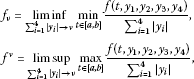where ν denote 0 or ∞.

To establish the existence of multiple positive solutions in E of problem (1.1), let us list the following assumptions, which will stand throughout the paper:

(H1) $f∈C(J× P 4 ,P)$, $h∈C(J,P)$ and

$∥ f ( t , y 1 , y 2 , y 3 , y 4 ) ∥ ≤a(t)+ ∑ i = 1 4 b i (t)∥ y i ∥,$
(2.3)

where $a(t)$ and $b i (t)$ are Lebesgue integrable functionals on J ($i=1,2,3,4$) and satisfying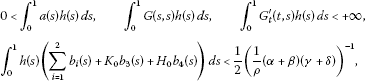(H2) $I k ∈C(P,P)$, $I ¯ k ∈C(P,P)$ and there exist positive constants $c k$, $c k ∗$ and $c ¯ k$ ($k=1,…,m$) satisfying

$∑ k = 1 m ( c k + c k ∗ + c ¯ k ) + 1 ρ (α+β) ∑ k = 1 m [ ( γ + δ ) ( c k ∗ + c ¯ k ) + γ c k ] < 1 4$
(2.4)

such that

$∥ I k ( y ) ∥ ≤ c k ∥y∥, ∥ I ¯ k ( y 1 , y 2 ) ∥ ≤ c k ∗ ∥ y 1 ∥+ c ¯ k ∥ y 2 ∥,∀t∈J,$

(H3) for any bounded set $B i ⊂E$, $i=1,2,3,4$, $f(t, B 1 , B 2 , B 3 , B 4 )$ and $I k ( B 1 )$ together with $I ¯ k ( B 1 , B 2 )$ are relatively compact sets,

(H4) $f 0 >m$,

(H5) $f ∞ , where

$m = max { ( σ ∫ a b G ( s , s ) h ( s ) d s ) − 1 , ( ∫ a b G t ′ ( t , s ) h ( s ) d s ) − 1 , 1 ρ ( α + β ) ( γ + δ ) ∫ 0 1 h ( s ) a ( s ) d s } .$
(2.5)

We shall reduce problem (1.1) to an integral equation in E. To this end, we first consider operator $A:K⟶PC[ J ¯ ,E]$ defined by

$( A y ) ( t ) = ∫ 0 1 G ( t , s ) h ( s ) f ( s , y ( s ) , y ′ ( s ) , ( T y ) ( s ) , ( S y ) ( s ) ) d s + ∑ 0 < t k < t [ I k ( y ( t k ) ) − ( t − t k ) I ¯ k ( y ( t k ) , y ′ ( t k ) ) ] + 1 ρ ( α t + β ) { ∑ k = 1 m ( γ ( 1 − t k ) + δ ) I ¯ k ( y ( t k ) , y ′ ( t k ) ) − γ ∑ k = 1 m I k ( y ( t k ) ) } .$
(2.6)

Lemma 2.1$y∈P C 1 [ J ¯ ,E]∩ C 2 [ J ′ ,E]$is a solution of problem (1.1) if and only if$y∈P C 1 [ J ¯ ,E]$is a solution of the following impulsive integral equation:

$y ( t ) = ∫ 0 1 G ( t , s ) h ( s ) f ( s , y ( s ) , y ′ ( s ) , ( T y ) ( s ) , ( S y ) ( s ) ) d s + ∑ 0 < t k < t [ I k ( y ( t k ) ) − ( t − t k ) I ¯ k ( y ( t k ) , y ′ ( t k ) ) ] + 1 ρ ( α t + β ) ∑ k = 1 m [ ( γ ( 1 − t k ) + δ ) I ¯ k ( y ( t k ) , y ′ ( t k ) ) − γ I k ( y ( t k ) ) ]$
(2.7)

i.e., y is a fixed point of operator A defined by (2.6) in$P C 1 [J,E]$.

Proof First suppose that $y∈P C 1 [J,E]$ is a solution of problem (1.1). It is easy to see by the integration of problem (1.1) that

$y ′ ( t ) = y ′ ( 0 ) − ∫ 0 t f ( s , y ( s ) , y ′ ( s ) , ( T y ) ( s ) , ( S y ) ( s ) ) d s + ∑ 0 < t k < t [ y ′ ( t k + ) − y ′ ( t k − ) ] = y ′ ( 0 ) − ∫ 0 t f ( s , y ( s ) , y ′ ( s ) , ( T y ) ( s ) , ( S y ) ( s ) ) d s − ∑ 0 < t k < t I ¯ k ( y ( t k ) , y ′ ( t k ) ) , ∀ t ∈ J .$
(2.8)

Integrate again, we get

$y ( t ) = y ( 0 ) + y ′ ( 0 ) t − ∫ 0 t ( t − s ) f ( s , y ( s ) , y ′ ( s ) , ( T y ) ( s ) , ( S y ) ( s ) ) d s + ∑ 0 < t k < t [ I k ( y ( t k ) ) − ( t − t k ) I ¯ k ( y ( t k ) , y ′ ( t k ) ) ] , ∀ t ∈ J .$
(2.9)

Letting $t=1$ in (2.8) and (2.9), we find that

$y ′ ( 1 ) = y ′ ( 0 ) − ∫ 0 1 f ( s , y ( s ) , y ′ ( s ) , ( T y ) ( s ) , ( S y ) ( s ) ) d s − ∑ k = 1 m I ¯ k ( y ( t k ) , y ′ ( t k ) ) .$
(2.10)
$y ( 1 ) = y ( 0 ) + y ′ ( 0 ) − ∫ 0 1 ( 1 − s ) f ( s , y ( s ) , y ′ ( s ) , ( T y ) ( s ) , ( S y ) ( s ) ) d s + ∑ k = 1 m [ I k ( y ( t k ) ) − ( 1 − t k ) I ¯ k ( y ( t k ) , y ′ ( t k ) ) ] .$
(2.11)

Since

$y ( 0 ) = β α y ′ ( 0 ) , y ( 1 ) = − δ γ { y ′ ( 0 ) − ∫ 0 1 f ( s , y ( s ) , y ′ ( s ) , ( T y ) ( s ) , ( S y ) ( s ) ) d s − ∑ k = 1 m I ¯ k ( y ( t k ) , y ′ ( t k ) ) } .$
(2.12)

We get

$y ′ ( 0 ) = α ρ { δ ∫ 0 1 f ( s , y ( s ) , y ′ ( s ) , ( T y ) ( s ) , ( S y ) ( s ) ) d s + γ ∫ 0 1 ( 1 − s ) f ( s , y ( s ) , y ′ ( s ) , ( T y ) ( s ) , ( S y ) ( s ) ) d s + ∑ k = 1 m [ ( γ ( 1 − t k ) + δ ) I ¯ k ( y ( t k ) , y ′ ( t k ) ) − γ I k ( y ( t k ) ) ] } , ∀ t ∈ J .$
(2.13)

Substituting (2.12) and (2.13) into (2.9), we obtain

$y ( t ) = 1 ρ ( α t + β ) { ∫ 0 1 ( γ ( 1 − s ) + δ ) f ( s , y ( s ) , y ′ ( s ) , ( T y ) ( s ) , ( S y ) ( s ) ) d s − ∑ k = 1 m [ γ I k ( y ( t k ) ) − ( γ ( 1 − t k ) + δ ) I ¯ k ( y ( t k ) , y ′ ( t k ) ) ] } − ∫ 0 t γ ( t − s ) f ( s , y ( s ) , y ′ ( s ) , ( T y ) ( s ) , ( S y ) ( s ) ) d s + ∑ 0 < t k < t [ I k ( y ( t k ) ) − ( t − t k ) I ¯ k ( y ( t k ) , y ′ ( t k ) ) ] = 1 ρ ∫ 0 t [ ( α t + β ) ( γ ( 1 − s ) + δ ) − ρ ( t − s ) ] f ( s , y ( s ) , y ′ ( s ) , ( T y ) ( s ) , ( S y ) ( s ) ) d s + 1 ρ ( α t + β ) ∫ t 1 ( γ ( 1 − s ) + δ ) f ( s , y ( s ) , y ′ ( s ) , ( T y ) ( s ) , ( S y ) ( s ) ) d s + ∑ 0 < t k < t [ I k ( y ( t k ) ) − ( t − t k ) I ¯ k ( y ( t k ) , y ′ ( t k ) ) ] + 1 ρ ( α t + β ) ∑ k = 1 m [ ( γ ( 1 − t k ) + δ ) I ¯ k ( y ( t k ) , y ′ ( t k ) ) − γ I k ( y ( t k ) ) ] = ∫ 0 1 G ( t , s ) h ( s ) f ( s , y ( s ) , y ′ ( s ) , ( T y ) ( s ) , ( S y ) ( s ) ) d s + ∑ 0 < t k < t [ I k ( y ( t k ) ) − ( t − t k ) I ¯ k ( y ( t k ) , y ′ ( t k ) ) ] + 1 ρ ( α t + β ) ∑ k = 1 m [ ( γ ( 1 − t k ) + δ ) I ¯ k ( y ( t k ) , y ′ ( t k ) ) − γ I k ( y ( t k ) ) ] .$

Conversely, if $y∈P C 1 [J,E]$ is a solution of the integral equation (2.7). Evidently, $Δy | t = t k = I k (y( t k ))$ ($k=1,…,m$). For $t= t k$, direct differentiation of the integral equation (2.7) implies

$y ′ ( t ) = 1 ρ { − γ ∫ 0 t ( α s + β ) f ( s , y ( s ) , y ′ ( s ) , ( T y ) ( s ) , ( S y ) ( s ) ) d s + α ∫ t 1 ( γ ( 1 − s ) + δ ) f ( s , y ( s ) , y ′ ( s ) , ( T y ) ( s ) , ( S y ) ( s ) ) d s − ∑ 0 < t k < t I ¯ k ( y ( t k ) , y ′ ( t k ) ) } − α ρ ∑ k = 1 m [ γ I k ( y ( t k ) ) − ( γ ( 1 − t k ) + δ ) I ¯ k ( y ( t k ) , y ′ ( t k ) ) ]$

and $y ′ ′ =−f(t,y(t), y ′ (t),(Ty)(t),(Sy)(t))$. So $y∈ C 2 [ J ′ ,E]$ and $−Δ y ′ | t = t k = I ¯ k (y( t k ), y ′ ( t k ))$ ($k=1,…,m$). It is easy to verify that $αy(0)−β y ′ (0)=0$ and $γy(1)+δ y ′ (1)=0$. The proof is complete. □

Thanks to (2.1), we know that

$G t ′ (t,s)= 1 ρ { − γ ( β + α s ) , 0 ≤ s ≤ t ≤ 1 , α ( γ + δ − γ s ) , 0 ≤ t ≤ s ≤ 1 .$

In the following, let $w ′ = sup t , s ∈ J , t ≠ s | G t ′ (t,s)|$. For $B⊂P C 1 [J,E]$, we denote $B ′ ={ y ′ : y ′ ∈B}⊂PC[J,E]$, $B(t)={y(t):y∈B}⊂E$ and $B ′ (t)={ y ′ (t): y ′ ∈B}⊂E$ ($t∈J$).

Lemma 2.2 ()

Let$D⊂P C 1 [J,E]$be a bounded set. Suppose that$D ′ (t)$is equi-continuous on each$J k$ ($k=1,…,m$). Then

$ϒ P C 1 (D)=max { sup t ∈ J ϒ ( D ( t ) ) , sup t ∈ J ϒ ( D ′ ( t ) ) } ,$

where ϒ and$ϒ P C 1$denote the Kuratowski measures of noncompactness of bounded sets in E and$P C 1 [J,E]$, respectively.

Lemma 2.3 ()

Let$H⊂PC[J,E]$be bounded equicontinuous, then$ϒ(H(t))$is continuous on J and

$ϒ ( { ∫ J y ( t ) d t : y ∈ H } ) ≤ ∫ J ϒ ( H ( t ) ) dt.$

Lemma 2.4 ()

$H⊂P C 1 [J,E]$is relatively compact if and only if each element$y(t)∈H$and$y ′ (t)∈H$are uniformly bounded and equicontinuous on each$J k$ ($k=1,…,m$).

Lemma 2.5 ()

Let E be a Banach space and$H⊂C[J,E]$if H is countable and there exists$φ∈L[J, R + ]$such that$∥y(t)∥≤φ(t)$, $t∈J$, $y∈H$. Then$ϒ({y(t):y∈H})$is integrable on J, and

$ϒ ( { ∫ J y ( t ) d t : y ∈ H } ) ≤2 ∫ J ϒ { y ( t ) : y ∈ H } dt.$

Lemma 2.6$A(K)⊂K$.

Proof For any $y∈K$, from Proposition 2.1 and (2.6), we obtain

$∥ A y ∥ ≤ ∫ 0 1 G ( s , s ) h ( s ) f ( s , y ( s ) , y ′ ( s ) , ( T y ) ( s ) , ( S y ) ( s ) ) d s + ∑ 0 < t k < t [ I k ( y ( t k ) ) − ( t − t k ) I ¯ k ( y ( t k ) , y ′ ( t k ) ) ] + 1 ρ ( α t + β ) { ∑ k = 1 m [ ( γ ( 1 − t k ) + δ ) I ¯ k ( y ( t k ) , y ′ ( t k ) ) ] − ∑ k = 1 m γ I k ( y ( t k ) ) } .$

On the other hand, for any $t∈[a,b]$, by (2.6) and Proposition 2.2, we know that

$A y ( t ) = ∫ 0 1 G ( t , s ) h ( s ) f ( s , y ( s ) , y ′ ( s ) , ( T y ) ( s ) , ( S y ) ( s ) ) d s + ∑ 0 < t k < t [ I k ( y ( t k ) ) − ( t − t k ) I ¯ k ( y ( t k ) , y ′ ( t k ) ) ] + 1 ρ ( α t + β ) ∑ k = 1 m [ ( γ ( 1 − t k ) + δ ) I ¯ k ( y ( t k ) , y ′ ( t k ) ) − γ I k ( y ( t k ) ) ] ≥ σ ∫ 0 1 G ( s , s ) h ( s ) f ( s , y ( s ) , y ′ ( s ) , ( T y ) ( s ) , ( S y ) ( s ) ) d s + ∑ 0 < t k < t [ I k ( y ( t k ) ) − ( t − t k ) I ¯ k ( y ( t k ) , y ′ ( t k ) ) ] + 1 ρ ( α t + β ) { ∑ k = 1 m [ ( γ ( 1 − t k ) + δ ) I ¯ k ( y ( t k ) , y ′ ( t k ) ) − γ I k ( y ( t k ) ) ] } ≥ σ ∥ A y ∥ .$

Hence, $A(K)⊂K$. □

Lemma 2.7 Suppose that (H1) and (H3) hold. Then$A:K⟶K$is completely continuous.

Proof Firstly, we show that $A:K⟶K$ is continuous. Assume that $y n , y 0 ∈K$ and $∥ y n − y 0 ∥⟶0$, $∥ y n ′ − y 0 ′ ∥⟶0$ ($n⟶∞$). Since $f∈C( J ¯ ×P×P×P×P,P)$, $I k ∈C(J,P)$ and $I ¯ k ∈C(J,P)$, then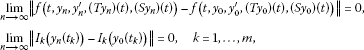(2.14)

and

$lim n ⟶ ∞ ∥ I ¯ k ( y n ( t k ) , y n ′ ( t k ) ) − I ¯ k ( y 0 ( t k ) , y 0 ′ ( t k ) ) ∥ =0,k=1,…,m.$
(2.15)

Thus, for any $t∈J$, from the Lebesgue dominated convergence theorem together with (2.14) and (2.15), we know that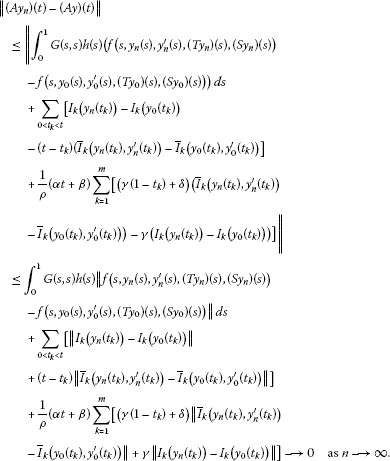Hence, $A:K⟶K$ is continuous.

Let $B⊂K$ be any bounded set, then there exists a positive constant $R 0 ∗$ such that $∥ y ∥ 1 ≤ R 0 ∗$. Thus, for any $y∈B$, $t∈(0,1)$, we know that

$| ( A y ) ′ ( t ) | = 1 ρ | { − γ ∫ 0 t ( α s + β ) h ( s ) f ( s , y ( s ) , y ′ ( s ) , ( T y ) ( s ) , ( S y ) ( s ) ) d s + α ∫ t 1 ( γ ( 1 − s ) + δ ) h ( s ) f ( s , y ( s ) , y ′ ( s ) , ( T y ) ( s ) , ( S y ) ( s ) ) d s } − ∑ 0 < t k < t I ¯ k ( y ( t k ) , y ′ ( t k ) ) + α ρ ∑ k = 1 m [ ( γ ( 1 − t k ) + δ ) I ¯ k ( y ( t k ) , y ′ ( t k ) ) − γ I k ( y ( t k ) ) ] | .$

Therefore,

$| ( A y ) ′ ( t ) | ≤ 1 ρ { − γ ∫ 0 t ( α s + β ) h ( s ) | f ( s , y ( s ) , y ′ ( s ) , ( T y ) ( s ) , ( S y ) ( s ) ) | d s + α ∫ t 1 ( γ ( 1 − s ) + δ ) h ( s ) | f ( s , y ( s ) , y ′ ( s ) , ( T y ) ( s ) , ( S y ) ( s ) ) | d s } + ∑ 0 < t k < t | I ¯ k ( y ( t k ) , y ′ ( t k ) ) | + α ρ ∑ k = 1 m [ ( γ ( 1 − t k ) + δ ) I ¯ k ( y ( t k ) , y ′ ( t k ) ) + γ | I k ( y ( t k ) ) | ] ≤ 1 ρ { − γ ∫ 0 t ( α s + β ) h ( s ) ( a ( s ) + ( ∑ i = 1 2 b i ( s ) + K 0 b 3 ( s ) + H 0 b 4 ( s ) ) ∥ y ∥ 1 ) d s + α ∫ t 1 ( γ ( 1 − s ) + δ ) h ( s ) ( a ( s ) + ( ∑ i = 1 2 b i ( s ) + K 0 b 3 ( s ) + H 0 b 4 ( s ) ) ∥ y ( s ) ∥ 1 ) d s } + ∑ k = 1 m ( c k ∗ + c ¯ k ) ∥ y ( s ) ∥ 1 + α ρ ∑ k = 1 m [ ( γ ( 1 − t k ) + δ ) ( c k ∗ + c ¯ k ) + γ c k ] ∥ y ( s ) ∥ 1 ≤ 1 ρ { − γ ∫ 0 t ( α s + β ) h ( s ) ( a ( s ) + ( ∑ i = 1 2 b i ( s ) + K 0 b 3 ( s ) + H 0 b 4 ( s ) ) R 0 ∗ ) d s + α ∫ t 1 ( γ ( 1 − s ) + δ ) h ( s ) ( a ( s ) + ( ∑ i = 1 2 b i ( s ) + K 0 b 3 ( s ) + H 0 b 4 ( s ) ) R 0 ∗ ) d s } + ∑ k = 1 m ( c k ∗ + c ¯ k ) R 0 ∗ + α ρ ∑ k = 1 m [ ( γ ( 1 − t k ) + δ ) ( c k ∗ + c ¯ k ) + γ c k ] R 0 ∗ ,$
(2.16)

$∀t∈J$, $t≠ t k$, $k=1,…,m$. Let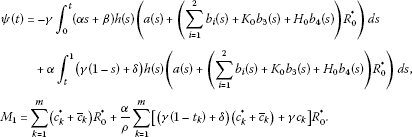Integrating $ψ(t)$ from 0 to 1 and exchanging integral sequence, then

$∫ 0 1 ψ ( t ) d t = ∫ 0 1 ∫ 0 s α ( γ + δ − γ s ) h ( s ) ( a ( s ) + ( ∑ i = 1 2 b i ( s ) + K 0 b 3 ( s ) + H 0 b 4 ( s ) ) R 0 ∗ ) d t d s − γ ∫ 0 1 ∫ s 1 ( β + α s ) h ( s ) ( a ( s ) + ( ∑ i = 1 2 b i ( s ) + K 0 b 3 ( s ) + H 0 b 4 ( s ) ) R 0 ∗ ) d t d s ≤ α ∫ 0 1 s ( γ + δ − γ s ) h ( s ) ( a ( s ) + ( ∑ i = 1 2 b i ( s ) + K 0 b 3 ( s ) + H 0 b 4 ( s ) ) R 0 ∗ ) d s − γ ∫ 0 1 ( β + α s ) h ( s ) ( a ( s ) + ( ∑ i = 1 2 b i ( s ) + K 0 b 3 ( s ) + H 0 b 4 ( s ) ) R 0 ∗ ) d s ≤ ρ ∫ 0 1 G ( s , s ) h ( s ) ( a ( s ) + ( ∑ i = 1 2 b i ( s ) + K 0 b 3 ( s ) + H 0 b 4 ( s ) ) R 0 ∗ ) d s < + ∞ .$
(2.17)

Thus, by (H1) and (2.17), we have $ψ(t)∈ L 1 (J)$. Hence, for any $0≤ t 1 ≤ t 2 ≤1$ and for all $y∈E$, from (2.16), we know that

$∥ ( A y ) ( t 1 ) − ( A y ) ( t 2 ) ∥ =| ∫ t 1 t 2 ( A y ) ′ (t)dt|≤ ∫ t 1 t 2 ( ψ ( t ) + M 1 ) dt.$
(2.18)

From (2.17), (2.18), and the absolutely continuity of integral function, we see that $A(B)$ is equicontinuous.

On the other hand, for any $y∈B$ and $t∈J$, we know that

$| ( A y ) ( t ) | = | ∫ 0 1 G ( t , s ) h ( s ) f ( s , y ( s ) , y ′ ( s ) , ( T y ) ( s ) , ( S y ) ( s ) ) d s + ∑ 0 < t k < t [ I k ( y ( t k ) ) − ( t − t k ) I ¯ k ( y ( t k ) , y ′ ( t k ) ) ] + 1 ρ ( α t + β ) { ∑ k = 1 m [ ( γ ( 1 − t k ) + δ ) I ¯ k ( y ( t k ) , y ′ ( t k ) ) − γ I k ( y ( t k ) ) ] } | ≤ ∫ 0 1 G ( s , s ) h ( s ) ( a ( s ) + ( ∑ i = 1 2 b i ( s ) + K 0 b 3 ( s ) + H 0 b 4 ( s ) ) R 0 ∗ ) d s + ∑ k = 1 m ( c k ∗ + c ¯ k ) R 0 ∗ ) + α ρ ∑ k = 1 m [ ( γ ( 1 − t k ) + δ ) ( c k ∗ + c ¯ k ) + γ c k ] R 0 ∗ < + ∞ .$

Therefore, $A(B)$ is uniformly bounded. By virtue of Lemma 2.3 and (H3), we know that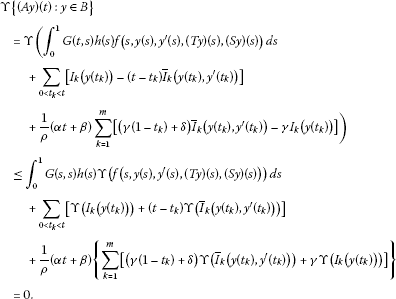So, $ϒ(A(B))=0$. Therefore, A is compact. To sum up, the conclusion of Lemma 2.7 follows. □

The main tools of the paper are the following well-known fixed-point index theorems (see ).

Lemma 2.8 Let$A:K→K$be a completely continuous mapping and$Ay≠y$for$y∈∂ K r$. Thus, we have the following conclusions:

1. (i)

If $∥y∥≤∥Ay∥$ for $y∈∂ K r$, then $i(A, K r ,K)=0$.

2. (ii)

If $∥y∥≥∥Ay∥$ for $y∈∂ K r$, then $i(A, K r ,K)=1$.

## 3 Main results

In this section, we establish the existence of positive solutions for problem (1.1) by making use of Lemma 2.8.

Theorem 3.1 Suppose that (H1)-(H4) hold. Then problem (1.1) has at least one positive solution.

Proof From (H4), there exists $ε>0$ such that $f 0 >m+ε$ and also there exists $r>0$ such that for any $0< ∑ i = 1 4 ∥ y i ∥≤r$ and $t∈[a,b]$, we have

$f(t, y 1 , y 2 , y 3 , y 4 )≥(m+ε) ∑ i = 1 4 ∥ y i ∥.$
(3.1)

Set $K r ={y∈K: ∥ y ∥ 1 . Then for any $y∈ K r ∩K$, by virtue of (3.1), we know that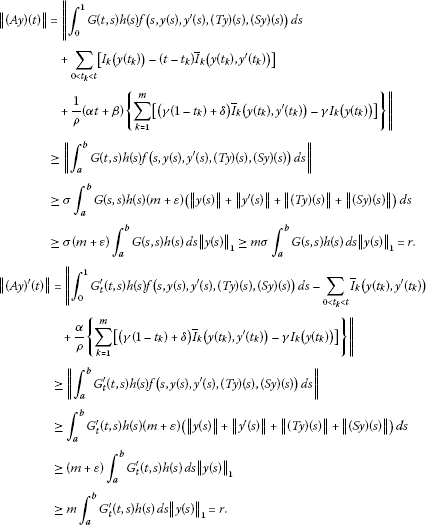So $∥ ( A y ) ( t ) ∥ 1 ≤r$. Therefore,

$i(A, K r ∩K,K)=0.$
(3.2)

Let $R>max{4m,2r}$. Then $K R$ is a bounded open subsets in E, and so for any $y∈ K R ∩K$ and $t∈J$, we obtain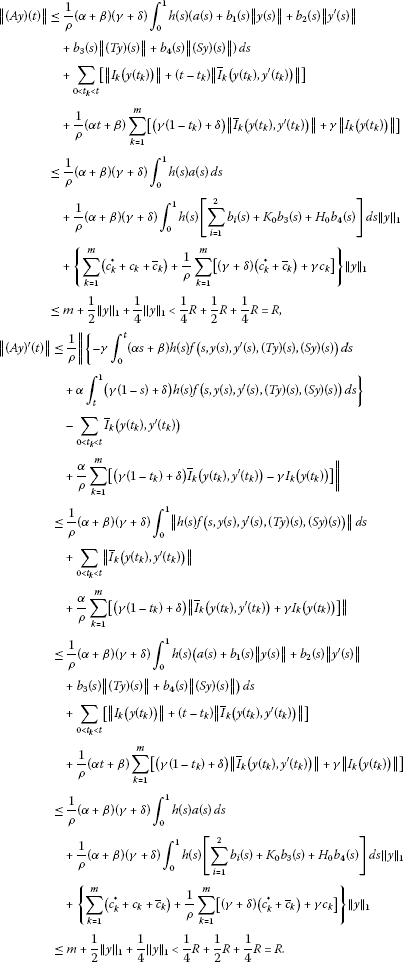Hence, $∥ A y ∥ 1 . Therefore,

$i(A, K R ∩K,K)=0.$
(3.3)

From (3.2) and (3.3), we get

$i ( A , ( K R ∩ K ) ∖ ( K ¯ r ∩ K ) , K ) =i(A, K R ∩K,K)−i(A, K r ∩K,K)=−1.$

Therefore, A has at least one fixed point on $( K R ∩K)∖( K ¯ r ∩K)$. Consequently, problem (1.1) has at least one positive solution. □

Theorem 3.2 Suppose that (H1)(H3) and (H5) are satisfied. Then problem (1.1) has at least one positive solution.

Proof From (H5), we can choose $ε 1 >0$ such that $f ∞ >m+ε$ and also there exists $R 1 >0$ such that for any $∑ i = 1 4 ∥ y i ∥≥ R 1$ and $t∈[a,b]$, we have

$f(t, y 1 , y 2 , y 3 , y 4 )≥(m+ ε 1 ) ∑ i = 1 4 ∥ y i ∥.$
(3.4)

Let $R ∗ > R 1 σ$. By virtue of (3.4), we know that

$f ( t , y ( t ) , y ′ ( t ) , ( T y ) ( t ) , ( S y ) ( t ) ) ≥(m+ ε 1 ) ( ∥ y ∥ + ∥ y ′ ∥ + ∥ T y ∥ + ∥ S y ∥ ) .$
(3.5)

Set $K R ∗ ={y∈K: ∥ y ∥ 1 < R ∗ }$. Then for any $y∈ K R ∗ ∩K$, by virtue of (3.5), we know that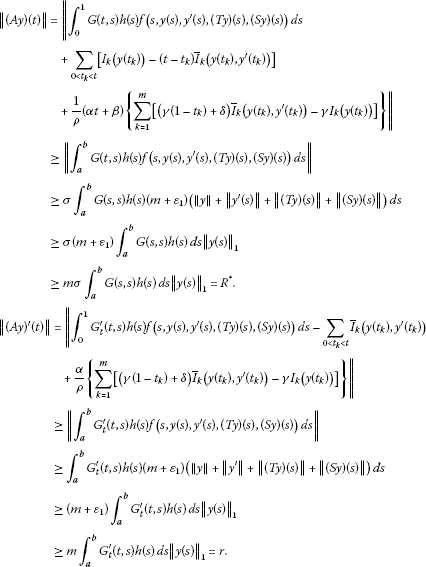So, $∥ ( A y ) ( t ) ∥ 1 ≤r$. Therefore,

$i(A, K R ∗ ∩K,K)=0.$
(3.6)

By the same method as the selection of $r ∗$ in Theorem 3.1, we can obtain $r ∗ < R ∗$ satisfying

$i(A, K r ∗ ∩K,K)=1.$
(3.7)

According to (3.6) and (3.7), we get

$i ( A , ( K R ∗ ∩ K ) ∖ ( K ¯ r ∗ ∩ K ) , K ) =i(A, K R ∗ ∩K,K)−i(A, K r ∗ ∩K,K)=−1.$

Therefore, A has at least one fixed point on $( K R ∗ ∩K)∖( K ¯ r ∗ ∩K)$. Consequently, problem (1.1) has at least one positive solution. The proof is complete. □

## 4 Concerned results and applications

In this section, we deal with a special case of the problem (1.1). The method is just similar to what we have done in Section 3, so we omit the proof of some main results of the section. Case $F(t,x(t), x ′ (t))=f(t,x(t), x ′ (t),(Ax)(t),(Bx)(t))$ is treated in the following theorem. Under the case, the problem (1.1) reduces to the following boundary value problems:

${ y ′ ′ ( t ) + h ( t ) F ( t , y ( t ) , y ′ ( t ) ) = θ , t ∈ J , t ≠ t k , Δ y | t = t k = I k ( y ( t k ) ) , − Δ y ′ | t = t k = I ¯ k ( y ( t k ) , y ′ ( t k ) ) , k = 1 , … , m , α y ( 0 ) − β y ′ ( 0 ) = 0 , γ y ( 1 ) + δ y ′ ( 1 ) = 0 ,$
(4.1)

where $F∈C(J×P×P,P)$, $h∈C(J)$.

Theorem 4.1 Assume that (H2) holds, and the following conditions are satisfied:

(C1) $F∈C(J× P 2 ,P)$, $h∈C(J,P)$and

$∥ F ( t , y 1 , y 2 ) ∥ ≤a(t)+ ∑ i = 1 2 b i (t)∥ y i ∥,$

where$a(t)$and$b i (t)$are Lebesgue integrable functionals on J ($i=1,2$) and satisfying(C2) for any bounded set$B i ⊂E$ ($i=1,2$), $F(t, B 1 , B 2 )$and$I k ( B 1 )$together with$I ¯ k ( B 1 , B 2 )$are relatively compact sets.

(C3) $lim ∥ y 1 ∥ + ∥ y 2 ∥ ⟶ 0 ∥ F ( t , y 1 , y 2 ) ∥ ∥ y 1 ∥ + ∥ y 2 ∥ >m$, where m is defined by (2.4). Then the problem (4.1) has at least one positive solution.

Theorem 4.2 Assume that (H2) and (C1)(C2) hold, and the following condition is satisfied:

(C4) $lim ∥ y 1 ∥ + ∥ y 2 ∥ ⟶ ∞ ∥ F ( t , y 1 , y 2 ) ∥ ∥ y 1 ∥ + ∥ y 2 ∥ >m$, where m is defined by (2.4). Then the problem (4.1) has at least one positive solution.

To illustrate how our main results can be used in practice, we present an example.

Example 4.1 Consider the following boundary value problem for scalar second-order impulsive integro-differential equation:

${ − y ′ ′ ( t ) = π sin t ( ln ( 3 + t 2 ) + t 3 y + t ∫ 0 t e − t s y d s + ∫ 0 1 e − 2 s y d s ) 720 t ( 1 + y + ∫ 0 t e − t s y ( s ) d s + ∫ 0 1 e − 2 s y ( s ) d s ) 2 , t ≠ t 1 , Δ y | t 1 = 1 3 = 1 120 y 3 ( 1 3 ) , − Δ y ′ | t 1 = 1 3 = 1 120 ( y 3 ( 1 3 ) + y ′ ( 1 3 ) ) ( y ( 1 3 ) + y ′ ( 1 3 ) ) 2 , α y ( 0 ) − β y ′ ( 0 ) = 0 , γ y ( 1 ) + δ y ′ ( 1 ) = 0 .$
(4.2)

## 5 Conclusion

The problem (4.2) has at least one positive solution $y ∗ (t)$.

For $t∈J$, $t≠ t 1$, let $h(t)= π 720 t$,

$F ( t , y , y ′ , ( T y ) ( t ) , ( S y ) ( t ) ) = sin t ( ln ( 3 + t 2 ) + t 3 y + t ∫ 0 t e − t s y d s + ∫ 0 1 e − 2 s y d s ) ( 1 + y + ∫ 0 t e − t s y ( s ) d s + ∫ 0 1 e − 2 s y ( s ) d s ) 2 .$

Choose $a(t)=sin t$. By simple computation, we know that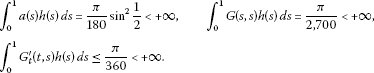Then conditions (H1)(H4) are satisfied. Therefore, by Theorem 3.1, the problem (4.2) has at least one positive solution.

Remark 5.1 In , by requiring that f satisfies some noncompact measure conditions and P is a normal cone, Guo established the existence of positive solutions for initial value problem. In the paper, we impose some weaker condition on f, we obtain the positive solution of the problem (1.1).

Remark 5.2 For the special case when the problem (1.1) has no singularities and $J=[0,a]$, our results still hold. Obviously, our theorems generalize and improve the results in .

## References

1. 1.

Wu CZ, Teo KL, Zhou Y, Yan WY: An optimal control problem involving impulsive integro-differential systems. Optim. Methods Softw. 2007, 22: 531-549. 10.1080/10556780601135688

2. 2.

Wu CZ, Teo KL, Zhou Y, Yan WY: Solving an identification problem as an impulsive optimal parameter selection problem. Comput. Math. Appl. 2005, 50: 217-229. 10.1016/j.camwa.2004.10.046

3. 3.

Guo D, Lakshmikantham V: Nonlinear Problems in Abstract Cones. Academic Press, Boston; 1988.

4. 4.

Guo D: Boundary value problems for impulsive integro-differential equations on unbounded domains in a Banach space. Appl. Math. Comput. 1999, 99: 1-15. 10.1016/S0096-3003(97)10174-6

5. 5.

Guo D: Existence of positive solutions for n th order nonlinear impulsive singular integro-differential equations in a Banach space. Nonlinear Anal. 2008, 68: 2727-2740. 10.1016/j.na.2007.02.019

6. 6.

Guo D: Existence of solutions for n th order impulsive integro-differential equations in Banach space. Nonlinear Anal. 2002, 44: 1339-1356.

7. 7.

Guo D: Extremal solutions for n th order impulsive integro-differential equations on the half-line in Banach space. Nonlinear Anal. 2006, 65: 677-696. 10.1016/j.na.2005.09.032

8. 8.

Guo D: Multiple positive solutions for n th order impulsive integro-differential equations in Banach space. Nonlinear Anal. 2005, 60: 955-976. 10.1016/j.na.2004.10.010

9. 9.

Liu L, Xu Y, Wu Y: On unique solution of an initial value problem for nonlinear first-order impulsive integro-differential equations of Volterra type in Banach spaces. Dyn. Contin. Discrete Impuls. Syst., Ser. A Math. Anal. 2006, 13: 641-652.

10. 10.

Xu Y: A global solutions of initial value problems for second order impulsive integro differential equations in Banach spaces. Acta Math. Sin., Chin. Ser. 2005, 25(1):47-56.

11. 11.

Liu L, Wu Y, Zhang X: On well-posedness of an initial value problem for nonlinear second order impulsive integro differential equations of Volterra type in Banach spaces. J. Math. Anal. Appl. 2006, 317: 634-649. 10.1016/j.jmaa.2005.12.032

12. 12.

Zhang X, Liu L: Initial value problem for nonlinear second order impulsive integro differential equations of mixed type in Banach spaces. Nonlinear Anal. 2006, 64: 2562-2574. 10.1016/j.na.2005.09.004

13. 13.

Guo D: Existence of solutions for n th-order impulsive integro-differential equations in a Banach space. Nonlinear Anal. 2001, 47: 741-752. 10.1016/S0362-546X(01)00219-X

14. 14.

Liu L, Wu C, Guo F: A unique solution of initial value problems for first order impulsive integro-differential equations of mixed type in Banach spaces. J. Math. Anal. Appl. 2002, 275: 369-385. 10.1016/S0022-247X(02)00366-9

15. 15.

Liu L: Iterative method for solution and coupled quasi-solutions of nonlinear integro differential equations of mixed type in Banach spaces. Nonlinear Anal. 2000, 42: 583-598. 10.1016/S0362-546X(99)00116-9

16. 16.

Liu L: The solutions of nonlinear integro-differential equations of mixed type in Banach spaces. Acta Math. Sin., Chin. Ser. 1995, 38: 721-731.

17. 17.

Zhang X, Feng M, Ge W: Existence of solutions of boundary value problems with integral boundary conditions for second-order impulsive integro-differential equations in Banach spaces. J. Comput. Appl. Math. 2010, 233: 1915-1926. 10.1016/j.cam.2009.07.060

18. 18.

Feng M, Pang H: A class of three-point boundary-value problems for second-order impulsive integro-differential equations in Banach spaces. Nonlinear Anal. 2009, 70: 64-82. 10.1016/j.na.2007.11.033

## Acknowledgements

The author is very grateful to Professor Lishan Liu and Professor R. P. Agarwal for their making many valuable comments. The author would like to express her thanks to the editor of the journal and the anonymous referees for their carefully reading of the first draft of the manuscript and making many helpful comments and suggestions which improved the presentation of the paper. The author was supported financially by the Foundation of Shanghai Municipal Education Commission (Grant Nos. DYL201105).

## Author information

Authors

### Corresponding author

Correspondence to Yan Sun.

### Competing interests

The author declares that she has no competing interests.

## Rights and permissions

Reprints and Permissions

Sun, Y. Positive solutions of Sturm-Liouville boundary value problems for singular nonlinear second-order impulsive integro-differential equation in Banach spaces. Bound Value Probl 2012, 86 (2012). https://doi.org/10.1186/1687-2770-2012-86